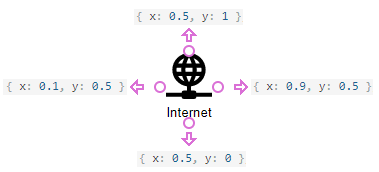# customShapes[]

An array of custom shapes.

Type:
Default Value: []

Use the customShapes option to extend a collection of built-in shapes with custom shapes. Custom shapes are displayed in the toolbox panel’s Custom tab.

##### jQuery
JavaScript
```\$(function() {
var diagram = \$("#diagram").dxDiagram({
customShapes: [{
id: 0,
title: "Internet",
svgUrl: "images/shapes/internet.svg",
svgLeft: 0.15,
svgTop: 0,
svgWidth: 0.7,
svgHeight: 0.7,
defaultWidth: 1.9,
defaultHeight: 1.9,
defaultText: "Internet",
allowHasText: true,
textLeft: 0,
textTop: 0.7,
textWidth: 1,
textHeight: 0.3,
connectionPoints: [
{ x: 0.5, y: 0 },
{ x: 0.9, y: 0.5 },
{ x: 0.5, y: 1 },
{ x: 0.1, y: 0.5 }
]
},
{
id: 1,
title: "Laptop",
svgUrl: "images/shapes/laptop.svg",
svgLeft: 0.15,
svgTop: 0,
svgWidth: 0.7,
svgHeight: 0.7,
defaultWidth: 1.9,
defaultHeight: 1.9,
defaultText: "Laptop",
allowHasText: true,
textLeft: 0,
textTop: 0.7,
textWidth: 1,
textHeight: 0.3,
connectionPoints: [
{ x: 0.5, y: 0 },
{ x: 0.9, y: 0.5 },
{ x: 0.5, y: 1 },
{ x: 0.1, y: 0.5 }
]
},
// ...
]
}).dxDiagram("instance");
});```

## allowHasText

Specifies whether a shape can contain text.

Type:

Boolean

## connectionPoints[]

An array of a shape's connection points.

Type:

##### jQuery
JavaScript
```\$(function() {
var diagram = \$("#diagram").dxDiagram({
customShapes: [{
id: 0,
connectionPoints: [
{ x: 0.5, y: 0 },
{ x: 0.9, y: 0.5 },
{ x: 0.5, y: 1 },
{ x: 0.1, y: 0.5 }
]
// ...```## defaultHeight

Specifies the initial height of a shape that is dragged from the toolbox to the diagram area.

Type:

Number

## defaultText

Specifies the initial text of a shape that is dragged from the toolbox to the diagram area.

Type:

String

## defaultWidth

Specifies the initial width of a shape that is dragged from the toolbox to the diagram area.

Type:

Number

## id

Specifies a shape's unique identifier.

Type:

Number

## svgHeight

Specifies the shape image's fractional height.

Type:

Number

This option specifies a fraction from 0 to 1 that represents the shape image height in relation to the shape height. If the svgHeight option is set to 1, the image height is equal to the shape height.

The absolute image height is calculated by multiplying the svgHeight value by the shape's height.

##### jQuery
JavaScript
```\$(function() {
var diagram = \$("#diagram").dxDiagram({
customShapes: [{
id: 0,
svgUrl: "images/shapes/internet.svg",
svgHeight: 0.7,
// ...```

## svgLeft

Specifies the shape image's left offset.

Type:

Number

This option specifies a fractional left offset of a shape image toward the shape width. If the svgLeft option is set to 0, the image's left edge matches the shape's left edge.

The absolute offset is calculated by multiplying the svgLeft value by the shape's width.

##### jQuery
JavaScript
```\$(function() {
var diagram = \$("#diagram").dxDiagram({
customShapes: [{
id: 0,
svgUrl: "images/shapes/internet.svg",
svgLeft: 0.15,
// ...```

## svgTop

Specifies the shape image's top offset.

Type:

Number

This option specifies the top offset (in fractions) of a shape's image in relation to the shape's height. If the svgTop option is set to 0, the image's top edge matches the shape's top edge.

The absolute offset is calculated by multiplying the svgTop value by the shape's height.

##### jQuery
JavaScript
```\$(function() {
var diagram = \$("#diagram").dxDiagram({
customShapes: [{
id: 0,
svgUrl: "images/shapes/internet.svg",
svgTop: 0,
// ...```

## svgUrl

Specifies a shape image's URL.

Type:

String

NOTE
Shape images should be supplied as SVG files.

## svgWidth

Specifies the shape image's fractional width.

Type:

Number

This option specifies a fraction from 0 to 1 that represents the shape image width in relation to the shape width. If the svgWidth option is set to 1, the image width is equal to the shape width.

The absolute image width is calculated by multiplying the svgWidth value by the shape width.

##### jQuery
JavaScript
```\$(function() {
var diagram = \$("#diagram").dxDiagram({
customShapes: [{
id: 0,
svgUrl: "images/shapes/internet.svg",
svgWidth: 0.7,
// ...```

## textHeight

Specifies the shape text container's height.

Type:

Number

This option specifies a fraction from 0 to 1 that represents the height of a shape's text in relation to the shape height. If the textHeight option is set to 1, the text height is equal to the shape height.

The absolute text height is calculated by multiplying the textHeight value by the shape height.

##### jQuery
JavaScript
```\$(function() {
var diagram = \$("#diagram").dxDiagram({
customShapes: [{
id: 0,
defaultText: "Internet",
textHeight: 0.3,
// ...```

## textLeft

Specifies the shape text's left offset.

Type:

Number

This option specifies the shape text's left offset (in fractions) in relation to the shape width. If the textLeft option is set to 0, the text's left edge matches the shape's left edge.

The absolute offset is calculated by multiplying the textLeft value by the shape width.

##### jQuery
JavaScript
```\$(function() {
var diagram = \$("#diagram").dxDiagram({
customShapes: [{
id: 0,
defaultText: "Internet",
textLeft: 0,
// ...```

## textTop

Specifies the shape text's top offset.

Type:

Number

This option specifies the shape text's top offset (in fractions) in relation to the shape height. If the textTop option is set to 0, the text's top edge matches the shape's top edge.

The absolute offset is calculated by multiplying the textTop value by the shape height.

##### jQuery
JavaScript
```\$(function() {
var diagram = \$("#diagram").dxDiagram({
customShapes: [{
id: 0,
defaultText: "Internet",
textTop: 0.7,
// ...```

## textWidth

Specifies the shape text container's width.

Type:

Number

This option specifies a fraction from 0 to 1 that represents the shape text's width in relation to the shape width. If the textWidth option is set to 1, the text width is equal to the shape width.

The absolute text width is calculated by multiplying the textWidth value by the shape width.

##### jQuery
JavaScript
```\$(function() {
var diagram = \$("#diagram").dxDiagram({
customShapes: [{
id: 0,
defaultText: "Internet",
textWidth: 1,
// ...```

## title

Specifies a shape's tooltip that is displayed in a toolbox panel.

Type:

String